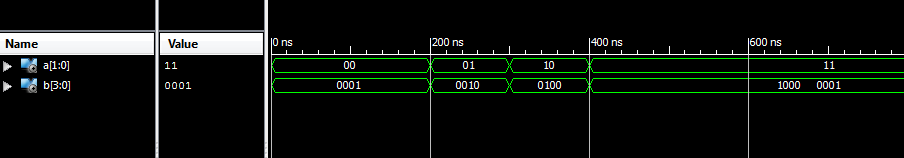# VHDL Code for 2 to 4 decoder

### Binary decoder

Binary decoder has n-bit input lines and 2 power n output lines. It can be 2-to-4, 3-to-8 and 4-to-16 line configurations. Binary decoder can be easily constructed using basic logic gates. VHDL Code of 2 to 4 decoder can be easily implemented with structural and behavioral modelling.### 2 to 4 Decoder design using logic gates### Truth Table for 2 to 4 DecoderSimilar to Encoder Design, VHDL Code for 2 to 4 decoder can be done in different methods like using case statement, using if else statement, using logic gates etc. Here we provide example code for all 3 method for better understanding of the language.

### VHDL Code for 2 to 4 decoder using case statement

```
library IEEE;
use IEEE.STD_LOGIC_1164.all;

entity decoder is
port(
a : in STD_LOGIC_VECTOR(1 downto 0);
b : out STD_LOGIC_VECTOR(3 downto 0)
);
end decoder;
architecture bhv of decoder is
begin

process(a)
begin
case a is
when "00" => b <= "0001"; when "01" => b <= "0010"; when "10" => b <= "0100"; when "11" => b <= "1000";
end case;
end process;

end bhv;

```

### VHDL Code for 2 to 4 decoder using if else statement

```library IEEE;
use IEEE.STD_LOGIC_1164.all;

entity decoder1 is
port(
a : in STD_LOGIC_VECTOR(1 downto 0);
b : out STD_LOGIC_VECTOR(3 downto 0)
);
end decoder1;

architecture bhv of decoder1 is
begin

process(a)
begin
if (a="00") then
b <= "0001";
elsif (a="01") then
b <= "0010";
elsif (a="10") then
b <= "0100";
else
b <= "1000";
end if;
end process;

end bhv;```

### VHDL Code for 2 to 4 decoder using logic gates

```library IEEE;
use IEEE.STD_LOGIC_1164.all;

entity decoder2 is
port(
a : in STD_LOGIC_VECTOR(1 downto 0);
b : out STD_LOGIC_VECTOR(3 downto 0)
);
end decoder2;

architecture bhv of decoder2 is
begin

b(0) <= not a(0) and not a(1);
b(1) <= not a(0) and a(1);
b(2) <= a(0) and not a(1);
b(3) <= a(0) and a(1);

end bhv;```

### TestBench VHDL Code for 2 to 4 decoder

```LIBRARY ieee;
USE ieee.std_logic_1164.ALL;

ENTITY tb_decoder IS
END tb_decoder;

ARCHITECTURE behavior OF tb_decoder IS

-- Component Declaration for the Unit Under Test (UUT)

COMPONENT decoder
PORT(
a : IN std_logic_vector(1 downto 0);
b : OUT std_logic_vector(3 downto 0)
);
END COMPONENT;

--Inputs
signal a : std_logic_vector(1 downto 0) := (others => '0');

--Outputs
signal b : std_logic_vector(3 downto 0);
-- appropriate port name

BEGIN

-- Instantiate the Unit Under Test (UUT)
uut: decoder PORT MAP (
a => a,
b => b
);

-- Stimulus process
stim_proc: process
begin
-- hold reset state for 100 ns.
wait for 100 ns;

a <= "00";

wait for 100 ns;

a <= "01";

wait for 100 ns;

a <= "10";

wait for 100 ns;

a <= "11";

wait;
end process;

END;
```

### TestBench waveform for 2 to 4 decoderThe above waveform displays the VHDL Code for 2 to 4 decoder implementation result.

### 8 thoughts on “VHDL Code for 2 to 4 decoder”

1.I think there’s a mistake in “VHDL Code for 2 to 4 decoder using logic gates” part. In 15th and 16th rows. Lines 15 and 16 need to be moved in each other. can you check it?

•Hi Yigit,
They are in right place.
A(0) represent LSB
A(1) represent MSB

2.3.here is a mistake in case stmnt ..

•when “00” its ans shuld be ….”0001″ ..bt u wrote “1000” bcz acc. to binary concept 1000 is 8

•Thanks for pointing out the mistake. Now it is corrected.

4.Nice!

•